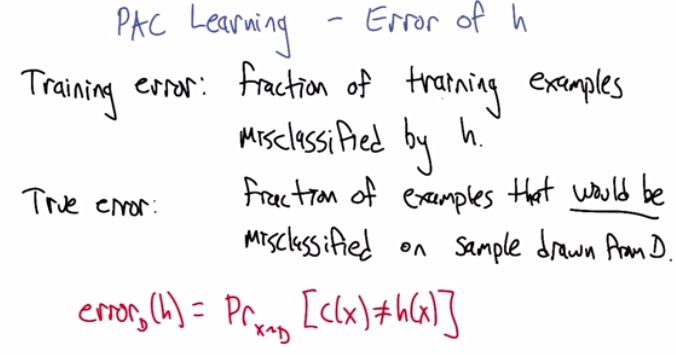• training error: the training example missclasfied by h (true hypothesis if got none)
• penalized if for the mistake in case our hypothesis is not the true hypothesis, but if it is, penalized get none
• true error is the error that missclassified by some hypothesis h. in this case the fault is in h. the best h, c, would get true error 0
• training error is some error/noise that happen in examples. no matter what the hypothesis are, even the best one would get incorrect. the fault is in the examples.
• the red one shows the probablity of error of some hypothesis, given that the probabilty of error if x from some distribution, in the case the hypothesis that we choose is not the correct hypothesis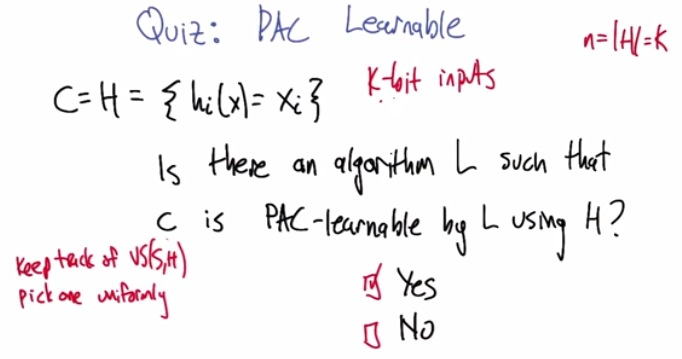• We have error goal, the probability that we achieve true hypothesis.
• and 1-delta, the probability that we are certain at least we get less than error.
• So we may not definitely correct, approximately may hard, but probably is.
• The C is PAC-learnable, if C has low error (high confidence) given set of parameters
• We can do that, if we keep track of of version space(the set of remaining possible inputs that determines the hypothesis)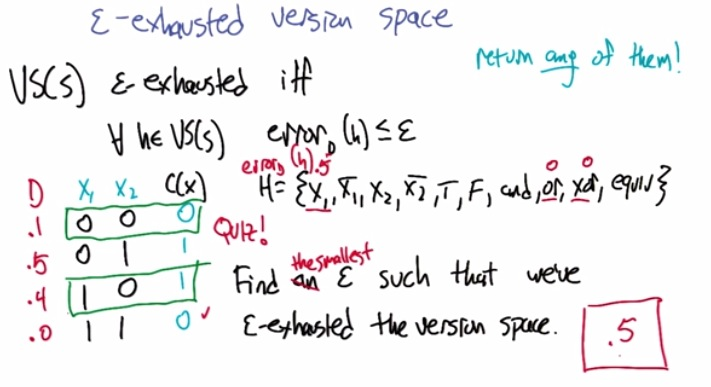• the version space must be e-exhausted iff all the hypothesis in the version space, its error below some threshold epsilon. If there's one hypothesis that's higher than epsilon, then it's still "epsilon-energized"
• For the case of the example, find epsilon such that it will act as a threshold that filter all the e-energized.
• The maximum will be 1, because the true hypothesis will not have true error(0), so filtering that definitely have done that.
• The version space earlier left with x1,or,xor. OR and XOR almost be the same, except last one. But since the probabilty of fourth is zero, meaning that the fourth case will never shows up , then OR is definitely no error.
• All that left is X1, since hypothesis X1 correctly guess first and third(prob equals 0.5) but wrong on second(0.5), that means X1 have the probability of missclasified which is 0.5.
• Since this still high error, and we want to filter that, the smallest is the same as X1 error, directly filter X1 and all that left is OR and XOR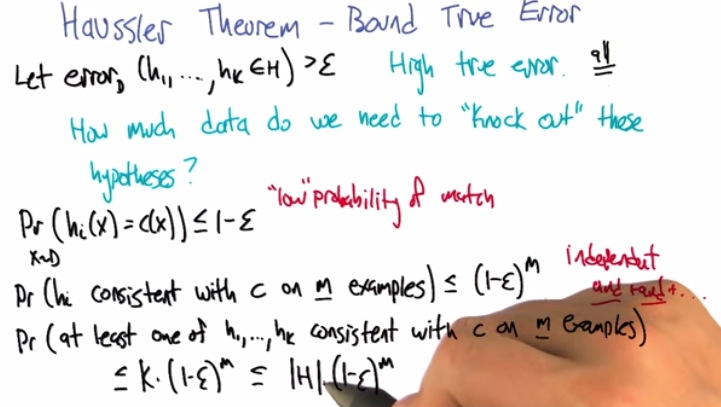• Haussler Theorem try to calculate the probability that we knock out all the hypothesis in the version space that have high true error. By high means the error is higher than epsilon threshold.
• First count the probabilty of hypothesis that equals true hypothesis, definitely lower than threshold, given one example.
• Next every m examples, the probabilty will be multiplied, over and over again, so that is (1-e)^m, given one particular hypothesis
• at least one consistent of having low error given all hypothesis. so K(hypothesis)x(1-e)^m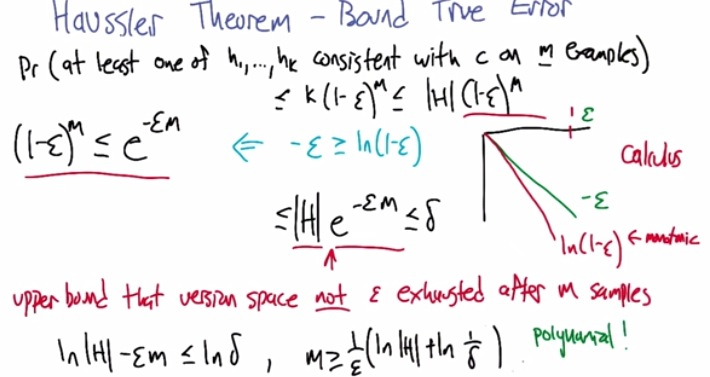• To complete in more mathematical examples, we're trying to find how much m, such that, we knock out all the high true error, given our tracked version space, and the threshold error that we are set.
• This slide takes last set of our probability, where we want to compute all hypothesis, at least one, consistent of our true hypothesis given n, below some threshold epsilon.
• So k(1-e)^m is, another definition, accumulate all hypothesis that have low error, given some threshold error, over m examples.
• The natural log (logn) is monotonic function, when decreasing, it will never increase. Can be static, but always decreasing.
• in magenta color, multiplied both side by e^(both side)*m  to be on the left side.
• Then put the result into set of hypothesis. left bottom will get to the right bottom if we separate all leaving just m, giving how much m would need to produce all hypothesis that below some very low error, with threshold(epsilon).
• The delta is just taking some certainty that the error higher than epsilon, otherwise all fails. All of the hypothesis in the version space doesn't matter.
• overall, we can say, how much samples that i would need to achieve the probabilty in which i'm very certain(delta) that at least one hypothesis of all my hypothesis in version space, bellow some error rate(epsilon)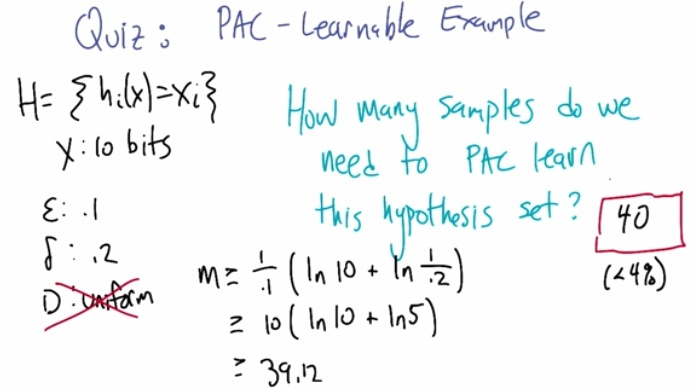• The input is 10 bits, 10 inputs, not 2^10 samples. Because all hypothesis directly handle the input.
• The distribution itself doesn't take into account. It can proceed all kind of distribution.
• So this is the sample which we are getting. Putting this and there we're getting at least 40 examples that all set of hypothesis, in the version space, have error rate of 10%.
• If for example we're having 1% error rate, than that would means we're gonna need 400 examples.